## Problem Solving Lesson 34 Convergence of three medians and three bisectors in a triangle (Chapter 9 Math 7 Connection) – Math Book

Problem Solving Lesson 34 Convergence of three medians and three bisectors in a triangle (Chapter 9 Math 7 Connection)

============

### Solution 9.20 page 76 Math textbook 7 Connecting knowledge volume 2 – KNTT

Given triangle ABC with two medians BN, CP and centroid G. Find the appropriate number in the question mark to get the equality:

BG = ? BN, CG = ? CP;

BG = ? GN, CG = ? GP.

Detailed explanation guide

Solution method

+) Use the theorem about the concurrency of the three medians of the triangle.

+) Rule for adding straight lines.

Detailed explanationSince G is the centroid of $$\Delta ABC$$ $$BG = \dfrac{2}{3}BN,CG = \dfrac{2}{3}CP$$

We have: GN = BN – BG = BN – $$\dfrac{2}{3}$$BN = $$\dfrac{1}{3}$$BN; GP = CP – CG = CP – $$\dfrac{2}{3}$$CP = $$\dfrac{1}{3}$$CP

Therefore, BN = 3. GN ; CP = 3. GP

Thus, $$BG = \dfrac{2}{3}BN = \dfrac{2}{3}.3.GN = 2GN;CG = \dfrac{2}{3}CP = \dfrac{2}{ 3}.3.GP = 2GP$$

So $$BG = \dfrac{2}{3}BN,CG = \dfrac{2}{3}CP$$;

BG = 2GN; CG = 2GP.

–>

— *****

### Solve problem 9.21 page 76 Math 7 Textbook Connecting knowledge volume 2 – KNTT

Prove that:

a) In an isosceles triangle, the two medians corresponding to the two sides are two equal line segments.

b) Conversely, if a triangle has two equal medians, then the triangle is isosceles.

Detailed instructions for solving problem 9.21

Solution method

Considering similar triangles, deduce the pair of corresponding sides that are congruent.

Detailed explanation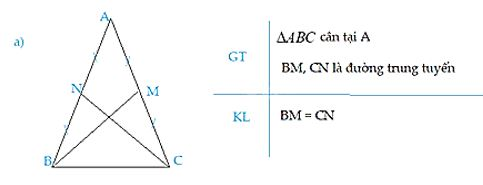Let BM and CN be the medians of $$\Delta ABC$$

$$\Rightarrow$$MA = MC = $$\dfrac{1}{2}$$AC; NA = NB = $$\dfrac{1}{2}$$AB

Since $$\Delta ABC$$ is balanced at A, AB = AC (property)

Therefore, AM = MC = NA = NB

Considering $$\Delta$$ANC and $$\Delta$$AMB, we have:

AN = AM

$$\widehat A$$ common

AC = AB

$$\Rightarrow$$$$\Delta$$ANC = $$\Delta$$AMB (cgc)

$$\Rightarrow$$ NC = MB ( 2 corresponding edges)

So the two medians corresponding to the two sides of an isosceles triangle are two equal line segments.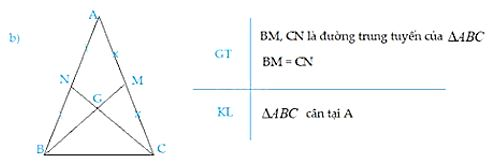Since $$∆ABC$$ has two medians $$BM$$ and $$CN$$ intersect at $$G$$

$$\Rightarrow$$ $$G$$ is the centroid of the triangle $$ABC$$.

$$\Rightarrow GB = \dfrac{2}{3}BM$$; $$GC = \dfrac{2}{3}CN$$ (property of medians in triangles)

Which $$BM = CN$$ (assumption) should $$GB = GC.$$

The triangle $$GBC$$ has $$GB = GC$$ so $$∆GBC$$ is isosceles at $$G$$.

$$\Rightarrow$$ $$\widehat{GCB} = \widehat{GBC}$$ (Property of isosceles triangle).

Consider $$∆BCN$$ and $$∆CBM$$ to have:

+) $$BC$$ is a common edge

+) $$CN = BM$$ (assumption)

+) $$\widehat{GCB} = \widehat{GBC}$$ (proved above)

Derive $$∆BCN = ∆CBM$$ (cgc)

$$\Rightarrow$$ $$\widehat{NBC} = \widehat{MCB}$$ (the two corresponding angles).

$$\Rightarrow ∆ABC$$ isosceles at $$A$$ (a triangle with two equal angles is an isosceles triangle)

–>

— *****

### Solve problem 9.22, page 76 Math 7 Textbook Connecting knowledge volume 2 – KNTT

Let the angle xOy be different from the flat angle. Use a compass to construct a circle with center O cutting Ox at A and intersecting Oy at B. Then construct two circles with center A and center B with equal radius so that they intersect at M and lie in the angle xOy. Prove that ray OM is the bisector of angle xOy.

Detailed instructions for solving problem 9.22

Solution method

Considering similar triangles, deduce pairs of corresponding angles that are congruent.

Detailed explanation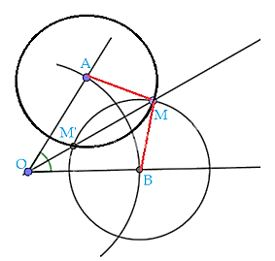We have: AM = radius of the circle with center A

BM = radius of the circle with center B

These two circles have the same radius

Therefore, AM = BM

Consider $$\Delta$$OAM and $$\Delta$$ONM have:

OA = OB( = radius of circle center O)

MA = MB

General OM

$$\Rightarrow$$ $$\Delta$$OAM and $$\Delta$$ONM ( ccc)

$$\Rightarrow$$ $$\widehat {AOM} = \widehat {BOM}$$ ( 2 corresponding angles)

Where OM lies between two rays OA and OB

$$\Rightarrow$$ OM is the bisector of angle AOB.

–>

— *****

### Solve problem 9.23 page 76 Math 7 Textbook Connecting knowledge volume 2 – KNTT

The symbol I is the point of convergence of the three bisectors of the triangle ABC. Calculate the angle BIC when the angle BAC is 120$$^\circ$$.

Detailed instructions for solving problem 9.23

Solution method

Apply the bisector of an angle and the sum of the 3 angles in a triangle is 180 degrees.

Detailed explanation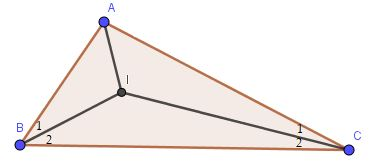Since BI is the bisector of angle ABC, $$\widehat {{B_1}} = \widehat {{B_2}} = \dfrac{1}{2}.\widehat {ABC}$$

Since CI is the bisector of angle ACB, $$\widehat {{C_1}} = \widehat {{C_2}} = \dfrac{1}{2}.\widehat {ACB}$$

Applying the triangle sum theorem in triangle ABC, we have:

$$\begin{array}{l}\widehat {BAC} + \widehat {ABC} + \widehat {ACB} = 180^\circ \\ \Rightarrow \widehat {ABC} + \widehat {ACB} = 180^ \circ – \widehat {BAC} = 180^\circ – 120^\circ = 60^\circ \\ \Rightarrow \widehat {{B_2}} + \widehat {{C_2}} = \dfrac{1}{2 }.\left( {\widehat {ABC} + \widehat {ACB}} \right) = \dfrac{1}{2}.60^\circ = 30^\circ \end{array}$$

Applying the theorem of the sum of three angles in triangle BIC, we have:

$$\begin{array}{l}\widehat {BIC} + \widehat {{B_2}} + \widehat {{C_2}} = 180^\circ \\ \Rightarrow \widehat {BIC} = 180^\circ – \left( {\widehat {{B_2}} + \widehat {{C_2}}} \right) = 180^\circ – 30^\circ = 150^\circ \end{array}$$

So $$\widehat {BIC} = 150^\circ$$

–>

— *****

### Solve problem 9.24 page 76 Math textbook 7 Connecting knowledge volume 2 – KNTT

Let BE and CF be the bisectors of an isosceles triangle ABC at A. Prove BE = CF.

Detailed instructions for solving problem 9.24

Solution method

Using the property of isosceles triangles, consider two congruent triangles and then indicate the two corresponding sides are congruent.

Detailed explanation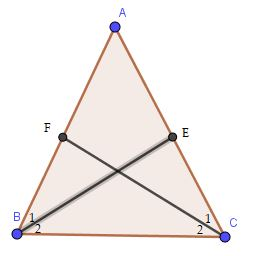Since triangle ABC is isosceles at A, AB = AC; $$\widehat {ABC} = \widehat {ACB}$$ (property)

Since BE is the bisector of angle ABC, $$\widehat {{B_1}} = \widehat {{B_2}} = \dfrac{1}{2}.\widehat {ABC}$$

Since CF is the bisector of angle ACB, $$\widehat {{C_1}} = \widehat {{C_2}} = \dfrac{1}{2}.\widehat {ACB}$$

Therefore, $$\widehat {{B_1}} = \widehat {{C_1}}$$

Considering $$\Delta ABE$$ and $$\Delta ACF$$, we have:

$$\widehat A$$ common

AB = AC

$$\widehat {{B_1}} = \widehat {{C_1}}$$

$$\Rightarrow \Delta ABE = \Delta ACF\left( {gcg} \right)$$

$$\Rightarrow$$BE = CF ( 2 corresponding edges)

–>

— *****

### Solve problem 9.25 on page 76 Math 7 Textbook Connecting knowledge volume 2 – KNTT

In triangle ABC, the bisectors of angles B and C intersect at D. Draw DP perpendicular to BC, DQ perpendicular to CA, DR perpendicular to AB.

a) Explain why DP = DR.

b) Explain why DP = DQ.

c) From sentences a and b, deduce DR = DQ. Why does D lie on the bisector of angle A? (This is one way of proving theorem 2).

Detailed instructions for solving problem 9.25

Solution method

Using the property of the bisector of an angle, consider 2 congruent triangles, infer the corresponding sides are congruent.

Detailed explanation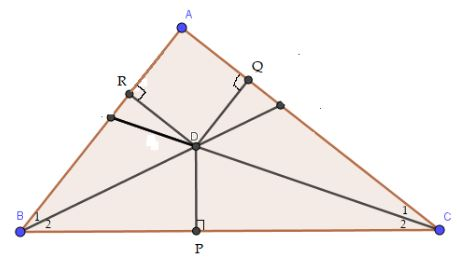a) Since BD is the bisector of angle ABC, $$\widehat {{B_1}} = \widehat {{B_2}} = \dfrac{1}{2}.\widehat {ABC}$$

Since CD is the bisector of angle ACB, $$\widehat {{C_1}} = \widehat {{C_2}} = \dfrac{1}{2}.\widehat {ACB}$$

Considering $$\Delta BDP$$ square at P and $$\Delta BDR$$ square at R, we have:

$$\widehat {{B_2}} = \widehat {{B_1}}$$

General BD

$$\Rightarrow \Delta BDP = \Delta BDR$$ ( hypotenuse – acute angle)

$$\Rightarrow$$ DP = DR ( 2 corresponding edges) (1)

b) Considering $$\Delta CDP$$ square at P and $$\Delta CDQ$$ square at Q, we have:

$$\widehat {{C_2}} = \widehat {{C_1}}$$

General CD

$$\Rightarrow \Delta CDP = \Delta CDQ$$ ( hypotenuse – acute angle)

$$\Rightarrow$$ DP = DQ ( 2 corresponding edges) (2)

c) From (1) and (2), we get: DR = DQ (same as DP).

D lies on the bisector of angle A since D is equidistant from AB and AC.

–>

— *****